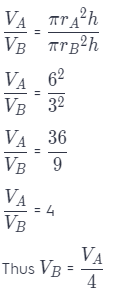# Texas Go Math Grade 8 Lesson 9.1 Answer Key Volume of Cylinders

Refer to our Texas Go Math Grade 8 Answer Key Pdf to score good marks in the exams. Test yourself by practicing the problems from Texas Go Math Grade 8 Lesson 9.1 Answer Key Volume of Cylinders.

## Texas Go Math Grade 8 Lesson 9.1 Answer Key Volume of Cylinders

Modeling the Volume of a Cylinder

A cylinder is a three-dimensional figure that has two congruent circular bases that lie in parallel planes. The volume of any three-dimensional figure is the number of cubic units needed to fill the space taken up by the solid figure.

One cube represents one cubic unit of volume. You can develop the formula for the volume of a cylinder using an empty soup can or other cylindrical container. First, remove one of the bases.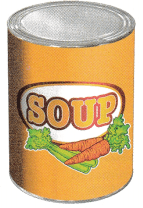A. Arrange centimeter cubes in a single layer at the bottom of the cylinder. Fit as many cubes into the layer as possible. How many cubes are in this layer?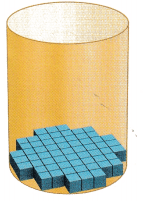B. To find how many layers of cubes fit in the cylinder, make a stack of cubes along the inside of the cylinder. How many layers fit in the cylinder?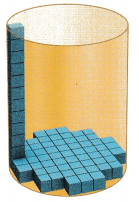C. How can you use what you know to find the approximate number of cubes that would fit in the cylinder?

Reflect

Question 1.
Make a Conjecture Suppose you know the area of the base of a cylinder and the height of the cylinder. How can you find the cylinder’s volume?
Volume of the cylinder is base times height. That is we will multiply the base area to the height of the cylinder, to find the volume
Volume of cylinder = base area × height

Question 2.
Let the area of the base of a cylinder be B and the height of the cylinder be h. Write a formula for the cylinder’s volume V.
Base area = B
Height = h
VoLume of cylinder = base area × height
V = Bh
V = r2πh

Example 1

Find the volume of each cylinder. Round your answers to the nearest tenth if necessary. Use 3.14 for π.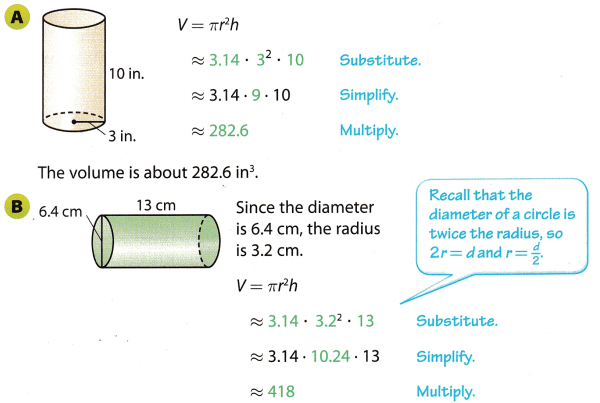The volume is about 418 cm3.

Reflect

Question 3.
What If? If you want a formula for the volume of a cylinder that involves the diameter d instead of the radius r, how can you rewrite it?
VoLume of cylinder = πr2h
Diameter(d) = 2r
r = $$\frac{d}{2}$$
Put the value of radius in the equation of voLume of a cylinder.
V = πr2h
V = π($$\frac{d}{2}$$)2h
V = π$$\frac{d^{2}}{4}$$h
V = $$\frac{\pi d^{2} h}{4}$$

Find the volume of each cylinder. Round your answers to the nearest tenth if necessary. Use 3.14 for π.

Question 4.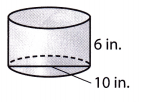Base diameter = 10 in
Base radius r = $$\frac{10}{2}$$
Base radius r = 5 in
Height = 6 in
VoLume of a cylinder = πr2h
V = 3.14 × 52 × 6
V = 3.14 × 25 × 6
V = 471 in3

Question 5.Base radius r = 4 ft
Length h = 12 ft
Volume of cylinder V = πr2h
V = 3.14 × 42 × 12
V = 3.14 × 16 × 12
V = 602.88 ft3
V ≈ 602.9 ft3

Question 6.
A drum company advertises a snare drum that is 4 inches high and 12 inches in diameter. Find the volume of the drum to the nearest tenth. Use 3.14 for π.
Diametar of the drum – d = 12 in
Radius of the drum – r = $$\frac{d}{2}$$ = $$\frac{12}{2}$$ = 6 in
Height – h = 4 in
VoLume of the drum – V = πr2h
V = 3.14 × 62 × 4
V = 3.14 × 36 × 4
V = 452.16 in3
V ≈ 452.2 in3

Question 1.
Vocabulary Describe the bases of a cylinder. (Explore Activity)
The two flat surfaces, on the ends of a cylinder, are the bases of the cylinder.

Question 2.
Figure 1 shows a view from above of inch cubes on the bottom of a cylinder. Figure 2 shows the highest stack of cubes that will fit inside the cylinder. Estimate the volume of the cylinder. Explain your reasoning. (Explore Activity)Number of inch cubes that fit in base of the cylinder = 61
Number of inch cubes that fit in length of the cylinder = 7
Volume of cylinder = base area × height
V = 61 × 7
V = 427 cubic units.
Volume of each cube = 1 in3
Volume of cylinder = 427 in3
The approximate volume of cylinder = 427 in3

Question 3.
Find the volume of the cylinder to the nearest tenth. Use 3.14 for π. (Example 1)The volume of the cylinder is approximately __________ m3.
V = πr2h
V = π ∙ 62 ∙ 15
V ≈ 3.14 × 36 × 15
V ≈ 1695.6m3

Question 4.
A Japanese odaiko is a very large drum that is made by hollowing out a section of a tree trunk. A museum in Takayama City has three odaikos of similar size carved from a single tree trunk. The largest measures about 2.7 meters in both diameter and length, and weighs about 4.5 metric tons. Using the volume formula for a cylinder, approximate the volume of the drum to the nearest tenth. (Example 2)
The volume of the drum is about ____________ m3.
Diameter of base of the drum = 2.7 m
Radius of the base of the drum = $$\frac{2.7}{2}$$
R = 1.35 m
Volume of cylinder = πR2h
Height (h) = 2.7 m
Volume = 3.14 × 1.352 × 2.7
V = 15.4511 m3
V ≈ 15.5 m3

Essential Question Check-In

Question 5.
How do you find the volume of a cylinder? Describe which measurements of a cylinder you need to know.
We need to find the radius of the base, r, and the height of the cylinder, h.
The volume of cylinder is = πr2h

Find the volume of each figure. Round your answers to the nearest tenth if necessary. Use 3.14 for π.

Question 6.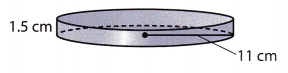Radius of base = 11 cm
Height of cylinder = 1.5 cm
Volume of cylinder = πr2h
V = 3.14 × 112 × 1.5
V = 56991
V ≈ 569.9 cm3
V = 569.9 cm32

Question 7.Radius of base = 4 in
Height of cylinder = 24 in
Volume of cylinder = πr2h
V = 3.14 × 42 × 24
V = 1205.76 in3
V ≈ 1205.8 in3

Question 8.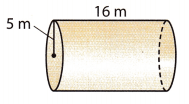Radius of base = 5 m
Height of cylinder = 16 m
Volume of cylinder = πr2h
V = 3.14 × 52 × 16
V = 1256 m3

Question 9.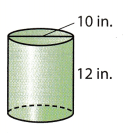Diameter of Base = 10 in
Radius of base = 5 in
Height of cylinder = 12 in
Volume of cylinder = πr2h
V = 3.14 × 52 × 12
V = 942 in3

Question 10.
A cylinder has a radius of 4 centimeters and a height of 40 centimeters.
Radius of base = 4 cm
Height of cylinder = 40 cm
Volume of cylinder = πr2h
V = 3.14 × 42 × 40
V = 2009.6 cm3

Question 11.
A cylinder has a radius of 8 meters and a height of 4 meters.
Radius of base = 8 m
Height of cylinder = 4 m
Volume of cylinder = πr2h
V = 3.14 × 82 × 4
V = 803.84 m3
V ≈ 803.8 m3

Round your answer to the nearest tenth, if necessary. Use 3.14 for π.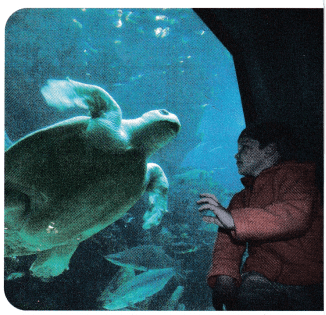Question 12.
The cylindrical Giant Ocean Tank at the New England Aquarium in Boston is 24 feet deep and has a radius of 18.8 feet. Find the volume of the tank.
Base radius of the tank = 18.8 ft
Depth of the tank in the ocean = 24 ft
Volume of the tank = πr2h
V = 3.14 × 18.82 × 24
V = 3.14 × 354.44 × 24
V = 26635.2384 ft3
V ≈ 26635.2 ft3

Question 13.
A standard-size bass drum has a diameter of 22 inches and is 18 inches deep. Find the volume of this drum.
Base diameter of a drum = 22 in
Base radius of the drum = $$\frac{22}{2}$$ in
Base radius of a drum = 11 in
Depth of the drum = 18 in
Volume of the drum = πr2h
V = 3.14 × 112 × 18
V = 3.14 × 121 × 18
V = 6838.92 in3
V ≈ 6838.9 in3

Question 14.
Grain is stored in cylindrical structures called silos. Find the volume of a silo with a diameter of 11.1 feet and a height of 20 feet.
Base diameter of silo 11.1 ft
Base radius of the silo = $$\frac{11.1}{2}$$ = 555 ft
Depth of the silo = 20 ft
Volume of the silo = πr2h
V = 3.14 × 5552 × 18
V = 3.14 × 30.8025 × 18
V = 1934.397 ft3
V = 1934.4 ft3

Question 15.
The Frank Erwin Center, or”The Drum:’ at the University of Texas in Austin can be approximated by a cylinder that is 120 meters in diameter and 30 meters in height. Find its volume.
Base diameter of a center 120 m
Base radius of the center = $$\frac{120}{2}$$ m
Base radius of the center = 60 m
Height of the center = 30 m
Volume of the center = πr2h
V = 3.14 × 602 × 30
V = 3.14 × 3600 × 30
V = 339120 m3

Question 16.
A barrel of crude oil contains about 5.61 cubic feet of oil. How many barrels of oil are contained in 1 mile (5280 feet) of a pipeline that has an inside diameter of 6 inches and is completely filled with oil? How much is “1 mile” of oil in this pipeline worth at a price of $100 per barrel? Answer: Volume of barrel = 5.61 cubic feet Length of the pipe = 1 mile = 5280 feet Diameter of the ripe = 6 inches = 0.5 feet Radius of the pipe = $$\frac{6}{2}$$ inches = 3 inches = 0.25 feet Volume of oil in the pipe = πr2h = 3.14 × 0.252 × 5280 = 1036.2 cubic feet Number of barrels in the pipe = $$\frac{1036.2}{5.61}$$ ≈ 184.7 barrels Cost of one barrel =$100
Cost of 184.7 barrels = 184.7 ∙ $100 =$18470

Question 17.
A pan for baking French bread is shaped like half a cylinder. It is 12 inches long and 3.5 inches in diameter. What is the volume of uncooked dough that would fill this pan?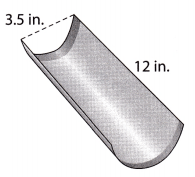The Length of the pan = 12 in
Diameter of the pan = 3.5 in
Radius = $$\frac{3.5}{2}$$
The volume of uncooked dough = Half the volume of full cylinder of above dimensions.
= $$\frac{\pi r^{2} h}{2}$$
= $$\frac{3.14 \times 1.75^{2} \times 12}{2}$$
= 57.697 in3
Volume of uncooked dough ≈ 57.7 in3

H.O.T. Focus on Higher Order Thinking

Question 18.
What’s the Error? A student said the volume of a cylinder with a 3-inch diameter is two times the volume of a cylinder with the same height and a 1.5-inch radius. What is the error?
To find the voLume of a cylinder we use the formula:
V = πr2h
If the diameter of the first cyLinder is 3 inches, it means that its radius is 1.5 inches. Therefore, its volume can be written as:
V1 = π ∙ (1.5)2 ∙ h
The volume of the second cylinder with the same height h and a radius of 15 inches is:
V2 = π ∙ (1.5)2 ∙ h
As we can see, the volumes of the two given cylinders are equal.
V1 = V2

Question 19.
Communicate Mathematical Ideas Explain how you can find the height of a cylinder if you know the diameter and the volume. Include an example with your explanation.
Let the diameter be D.
Radius r = $$\frac{D}{2}$$
Volume = πr2h
Volume = π($$\frac{D}{2}$$)2h
V = π$$\frac{D^{2}}{4}$$h
h = $$\frac{4 V}{\pi D^{2}}$$
To find the height of a cylinder with diameter D = 2m
Let the volume be 10 m 3
h = $$\frac{4 V}{\pi D^{2}}$$
h = $$\frac{4 \times 10}{3.14 \times 2^{2}}$$
h = 3.18 m 3

Question 20.
Analyze Relationships Cylinder A has a radius of 6 centimeters. Cylinder B has the same height and a radius half as long as cylinder A. What fraction of the volume of cylinder A is the volume of cylinder B? Explain.